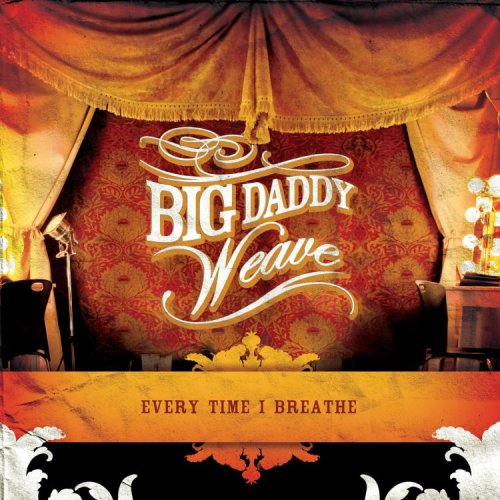### ChristianPowerPraise.Net Song InfoEvery Time I Breathe (2006)
Label: Fervent

Request this song
Let It Rise by Big Daddy Weave

Lyrics
Let the glory of the Lord rise among us
Let the glory of the Lord rise among us
Let the praises of the King rise among us
Let it rise

Let the songs of the Lord rise among us
Let the songs of the Lord rise among us
Let the joy of the King rise among us
Let it rise

Oh, Oh, Oh
Let it rise

Let the glory of the Lord rise among us
Let the glory of the Lord rise among us
Let the praises of the King rise among us
Let it rise

Let the songs of the Lord rise among us
Let the songs of the Lord rise among us
Let the joy of the King rise among us
Let it rise

(2x's)
Oh, Oh, Oh
Let it rise

(4x's)
Let it rise

Let the glory of the Lord rise among us
Let the glory of the Lord rise among us
Let the praises of the King rise among us
Let it rise

Let the songs of the Lord rise among us
Let the songs of the Lord rise among us
Let the joy of the King rise among us
Let it rise

(3x's)
Oh, Oh, Oh
Let it rise

(10x's)
Let it rise